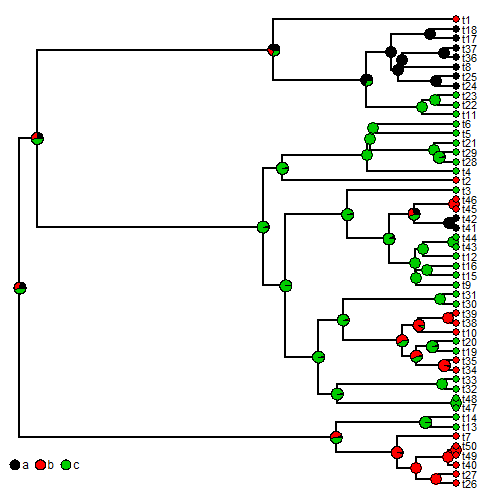## Monday, March 21, 2016

### New function and method updates for objects of class `"simmap"` and `"multiSimmap"`

I just uploaded a very small update to phytools which now adds the aliases & S3 method definitions to the documentation pages of `plotSimmap` and `describe.simmap`.

This just now allows users to search for help using, for instance:

``````help(summary.simmap)
## or
?plot.multiSimmap
``````

Here's a quick demo reminder of some of the uses of these functions:

``````library(phytools)
tree
``````
``````##
## Phylogenetic tree with 50 tips and 49 internal nodes.
##
## Tip labels:
##  t26, t27, t40, t49, t50, t7, ...
##
## Rooted; includes branch lengths.
``````
``````x
``````
``````## t26 t27 t40 t49 t50  t7 t13 t14 t47 t48 t32 t33 t34 t35 t19 t20 t10 t38
##   b   b   b   b   b   b   c   c   c   c   c   c   b   b   c   c   b   b
## t39 t30 t31  t9 t15 t16 t12 t43 t44 t41 t42 t45 t46  t3  t2  t4 t28 t29
##   b   c   c   c   c   c   c   c   c   a   a   b   b   c   b   c   c   c
## t21  t5  t6 t11 t22 t23 t24 t25  t8 t36 t37 t17 t18  t1
##   c   c   c   c   c   c   a   a   a   a   a   a   a   b
## Levels: a b c
``````
``````mapped.trees<-make.simmap(tree,x,model="ER",nsim=100)
``````
``````## make.simmap is sampling character histories conditioned on the transition matrix
##
## Q =
##            a          b          c
## a -1.4139675  0.7069837  0.7069837
## b  0.7069837 -1.4139675  0.7069837
## c  0.7069837  0.7069837 -1.4139675
## (estimated using likelihood);
## and (mean) root node prior probabilities
## pi =
##         a         b         c
## 0.3333333 0.3333333 0.3333333
``````
``````## Done.
``````
``````mapped.trees
``````
``````## 100 phylogenetic trees with mapped discrete characters
``````
``````## summary
obj<-summary(mapped.trees)
obj
``````
``````## 100 trees with a mapped discrete character with states:
##  a, b, c
##
## trees have 12.59 changes between states on average
##
## changes are of the following types:
##       a,b  a,c b,a  b,c  c,a  c,b
## x->y 1.38 1.67 0.9 2.25 1.86 4.53
##
## mean total time spent in each state is:
##              a         b         c    total
## raw  1.5539349 2.1136781 5.2920042 8.959617
## prop 0.1734376 0.2359117 0.5906507 1.000000
``````
``````class(obj)
``````
``````##  "describe.simmap"
``````
``````## plot method for summary
plot(obj,fsize=0.9)
colnames(obj\$ace)),prompt=FALSE,
x=0,y=3,vertical=FALSE,shape="circle")
``````I also added the simple, but overdue, function `mapped.states` which returns a vector (for a single mapped tree), matrix (for a set of mapped trees in which each tree has the same number of mapped states), or list (for the most general case) containing the set of states that are mapped on the tree. Code for this function is as follows:

``````mapped.states<-function(tree,...){
if(!(inherits(tree,"simmap")||inherits(tree,"multiSimmap")))
stop("tree should be an object of class \"simmap\" or \"multiSimmap\".")
else {
if(inherits(tree,"simmap")){
obj<-sort(unique(c(getStates(tree,type="nodes"),
getStates(tree,type="tips"))))
} else {
X<-rbind(getStates(tree,type="nodes"),getStates(tree,
type="tips"))
obj<-apply(X,2,function(x) sort(unique(x)))
}
}
obj
}
``````

It will henceforward be part of the namespace for phytools.

This package version can be installed from GitHub using devtools as follows:

``````library(devtools)
install_github("liamrevell/phytools")
``````

That's it.